International
Tables for
Crystallography
Volume B
Reciprocal space
Edited by U. Shmueli

International Tables for Crystallography (2006). Vol. B. ch. 2.4, pp. 272-273   | 1 | 2 |

## Section 2.4.4.5. Use of anomalous scattering in phase evaluation

M. Vijayana* and S. Ramaseshanb

aMolecular Biophysics Unit, Indian Institute of Science, Bangalore 560 012, India, and bRaman Research Institute, Bangalore 560 080, India
Correspondence e-mail:  mv@mbu.iisc.ernet.in

#### 2.4.4.5. Use of anomalous scattering in phase evaluation

| top | pdf |

When anomalous-scattering data have been collected from derivative crystals,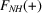and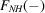can be formally treated as arising from two independent derivatives. The corresponding Harker diagram is shown in Fig. 2.4.4.4. Thus, in principle, protein phase angles can be determined using a single derivative when anomalous-scattering effects are also made use of. It is interesting to note that the information obtained from isomorphous differences,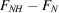, and that obtained from anomalous differences,, are complementary. The isomorphous difference for any given reflection is a maximum whenandare parallel or antiparallel. The anomalous difference is then zero, if all the anomalous scatterers are of the same type, and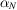is determined uniquely on the basis of the isomorphous difference. The isomorphous difference decreases and the anomalous difference increases as the inclination betweenandincreases. The isomorphous difference tends to be small and the anomalous difference tends to have the maximum possible value whenandare perpendicular to each other. The anomalous difference then has the predominant influence in determining the phase angle.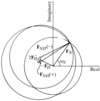Figure 2.4.4.4 | top | pdf |Harker construction using anomalous-scattering data from a single derivative.

Although isomorphous and anomalous differences have a complementary role in phase determination, their magnitudes are obviously unequal. Therefore, whenandare treated as arising from two derivatives, the effect of anomalous differences on phase determination would be only marginal as, for any given reflection,is usually much smaller than. However, the magnitude of the error in the anomalous difference would normally be much smaller than that in the corresponding isomorphous difference. Firstly, the former is obviously free from the effects of imperfect isomorphism. Secondly,andare expected to have the same systematic errors as they are measured from the same crystal. These errors are eliminated in the difference between the two quantities. Therefore, as pointed out by North (1965), the r.m.s. error used for anomalous differences should be much smaller than that used for isomorphous differences. Denoting the r.m.s. error in anomalous differences by E′, the new expression for the probability distribution of protein phase angle may be written as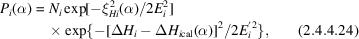where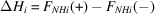and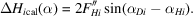Here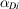is the phase angle of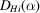[see (2.4.4.19) and Fig. 2.4.4.2].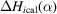is the anomalous difference calculated for the assumed protein phase angle.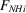may be taken as the average of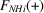and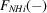for calculating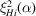using (2.4.4.20).

### ReferencesNorth, A. C. T. (1965). The combination of isomorphous replacement and anomalous scattering data in phase determination of non-centrosymmetric reflexions. Acta Cryst. 18, 212–216.Google Scholar